#### Introduction

When student compounded preparations are analyzed, a standard curve is constructed by making the same preparation with varying concentrations of the API. The purpose of this investigation was to examine different standard curves to evaluate how linearity is affected by the inclusion of (0, 0) as the first data point. The question was raised because when using spectrophotometers for the analysis, the instrument must be “zeroed” which might define a valid point to add to the curve. But when using HPLC, no such “zeroing” occurs. While analyzing standard curves for several different preparations, we noticed that some were straight lines while others produced curved lines. These differences appear to be dependent on the wavelength and the compound being analyzed. Another purpose of this experiment was to see how standard curves are affected when analyzed using different wavelengths of light in the spectrophotometer.

The standard curves analyzed in this study compare absorbance with solutions of increasing concentrations of diphenhydramine (DPH) or potassium permanganate (KMnO4). The concentrations of the compound-containing solutions are measured using a “blank” containing the solution without the compound is used to zero the spectrophotometer.

#### Materials

• 150 mL beaker
• Electronic prescription balance
• Stir bar
• 4 oz. amber plastic prescription bottle with tight closure
• Spectrophotometer
• Glass cuvettes
Diphenhydramine Syrup
Diphenydramine HCl 250 mg
Glycerin 5 mL
Syrup NF 30 mL (85 g sucrose per 100 mL water)
Vanillin Alcoholic Solution 67 mg% 0.2 mL
Distilled water qs 100 mL

#### Method of Preparation per Formulation Record

1. Calibrate an amber plastic prescription bottle to the final volume.
2. Using the torsion balance, weight the diphenhydramine HCl. (Electronic balance was used for this instead of torsion balance)
3. Transfer the diphenhydramine HCl into a 150 ml beaker.
4. Using a bottle-to-syringe adapter, measure and transfer glycerin into the beaker.
5. Using a bottle-to-syringe adapter, measure and transfer vanillin solution into the beaker.
6. Using a graduated cylinder, measure and transfer the simple syrup into the beaker.
7. Add a portion of water to the beaker, add a stirring bar, and stir the mixture gently until solution occurs.
8. Transfer the mixture into the calibrated prescription bottle.
9. Rinse the beaker with portions of water, adding to the prescription bottle.
10. Bring the solution to final volume. Shake well to mix.

Source of Recipe: Pharmlabs Website (pharmlabs.unc.edu)

Literature Information: Diphenhydramine hydrochloride: stable under ordinary conditions; slowly darkens on exposure to light (Merck Index, p. 561, 12th edition)
The above formulation produces a solution with a diphenhydramine concentration of 2.5 mg/mL. To make a set of standards, the above method of preparation was followed with the exception of using different amounts of diphenhydramine. Final concentrations and amounts of diphenhydramine are shown in Table 1.

 Strength (mg/mL) Diphenhydramine (mg) 0 0 mg 0.5 50 mg 1 100 mg 1.5 150 mg 2 200 mg 2.5 250 mg 3 300 mg 3.5 350 mg

#### DPH Standard Solutions in Glass Cuvettes

Approximately 2 mL of each solution was placed in a glass cuvette. A blank containing no DPH was used to zero the spectrophotometer. The absorbance was measured using a spectrophotometer at wavelengths of 265 nm, 270 nm, and 275 nm. Absorbance results are listed in Table 2.

 Strength (mg/mL) Absorbance at 265 nm Absorbance at 270 nm Absorbance at 275 nm 0 0 0 0 0.5 0.538 0.25 0.039 1 1.033 0.471 0.079 1.5 1.456 0.648 0.121 2 1.717 0.784 0.147 2.5 1.867 0.905 0.178 3 1.955 1.041 0.221 3.5 1.966 1.099 0.232

Below are graphs of the concentrations vs absorbance (Figures 1, 2, and 3). The first graph shown for each wavelength includes (0, 0) as a data point, while the second does not include (0, 0). R2 values are included to show how the inclusion/exclusion of (0, 0) affects the linearity of the standard curve.

 Figure 1. Concentration of DPH vs Absorbance of DPH Solutions at λ=265 nm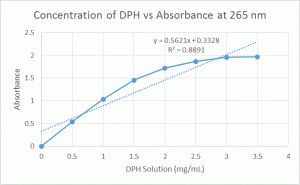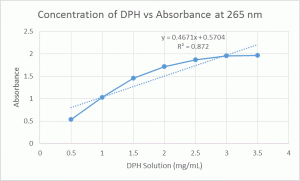Figure 2. Concentrations of DPH vs Absorbance of DPH Solutions at λ=270 nm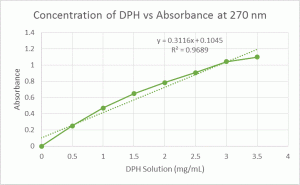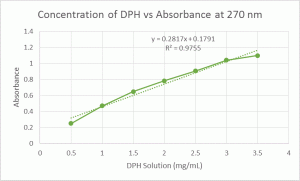Figure 3. Concentration of DPH vs Absorbance of DPH Solutions at λ=275 nm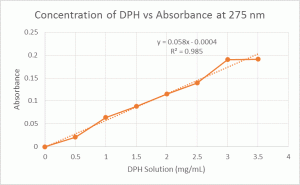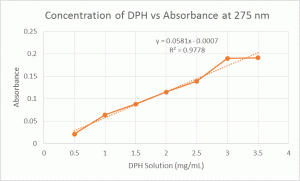#### DPH and Water

To determine if any of the other ingredients were interfering with absorbance, a formulation consisting only of DPH and water was made. These solutions had the same concentrations of DPH as the previous trial, but concentrations of 0.125 mg/mL and 0.25 mg/mL (containing 12.5 mg and 25 mg diphenhydramine, respectively) were added for more data points. Absorbance values are listed in Table 3.

#### DPH Solutions

 Strength (mg/mL) Absorbance at 265 Absorbance at 270 Absorbance at 275 0 0 0 0 0.125 0.153 0.071 0.013 0.25 0.278 0.126 0.022 0.5 0.538 0.237 0.04 1 1.033 0.436 0.075 1.5 1.443 0.603 0.108 2 1.68 0.77 0.141 2.5 1.843 0.907 0.175 3 1.895 1.016 0.203 3.5 1.916 1.111 0.23

Below are graphs of the concentrations vs absorbance (Figures 4, 5, and 6). The first graph shown for each wavelength includes (0, 0) as a data point, while the second does not include (0, 0). R2 values are included to show how the inclusion/exclusion of (0, 0) affects the linearity of the standard curve.

 Figure 4. Concentration of DPH in Water vs Absorbance of DPH Solutions at λ=265 nm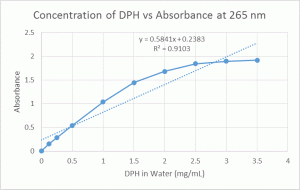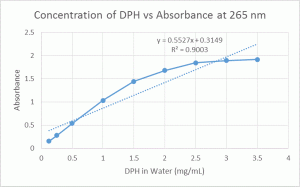Figure 5. Concentration of DPH in Water vs Absorbance of DPH Solutions at λ=270 nm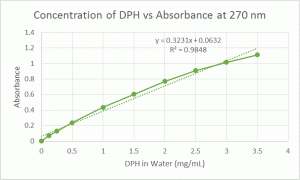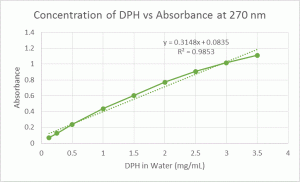Figure 6. Concentration of DPH in Water vs Absorbance of DPH Solutions at λ=275 nm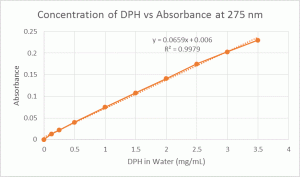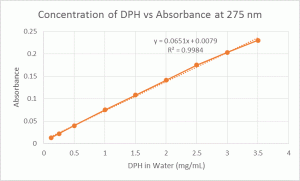After 2 weeks, visible microbe growth could be seen in some of the solutions. The blank had the most microbe growth (slightly cloudy with visible microbe colonies), the 0.125 mg/mL solution had less growth (some visible colonies), and the 0.25 mg/mL solution had the least amount of growth (few visible colonies). All other solutions contained no visible microbe growth. Though used primarily as an antihistamine, DPH also possesses antimicrobial effects in vitro. Studies have shown that DPH has bacteriostatic and bactericidal effects, which are dose-dependent (Güzelant, et al.)

#### Materials

• Scintillation vials (7)
• 1 × 10-3 M KMnO4 solution (MW KMnO4 = 158.03)
• Distilled water
• Methacrylate cuvettes
• Spectrophotometer

#### Method of Preparation:

1. Combine KMnO4 solution and water in the scintillation vials, using the volumes listed in Table 4. Label each vial with the appropriate concentration.
2. Set spectrophotometer wavelength at 500 nm. Place approximately 2 mL of distilled water in a cuvette and zero the spectrophotometer.
3. Place approximately 2 mL of each KMnO4 solution in a cuvette and measure absorbance.
4. Repeat steps 2-3 using wavelength at 530 nm and 550 nm.
 Strength (mg/mL) Distilled Water (mL) Concentraition of KMnO4 (M) 0 20 0 0.25 19.75 1.25 × 10-5 0.5 19.5 2.5 × 10-5 1 19 5 × 10-5 2 18 1 × 10-4 4 16 2 × 10-4 10 10 5 × 10-4
 Concentraition of KMnO4 (M) Absorbance at 500 Absorbance at 530 Absorbance at 550 0 0 0 0 1.25 × 10-5 0.021 0.031 0.029 2.5 × 10-5 0.039 0.057 0.054 5 × 10-5 0.077 0.115 0.107 1 × 10-4 0.153 0.227 0.213 2 × 10-4 0.305 0.453 0.424 5 × 10-4 0.755 1.122 1.049

Below are graphs of the concentrations vs absorbance (Figures 7, 8, and 9). All graphs contain (0, 0) as a data point, and R2 values are included. The graphs of concentration vs absorbance which did not include (0, 0) as a data point were not included since they were also completely linear, but the linear equation and R2 value for these are listed next to each graph for comparison.

 Figure 7. Concentration of KMnO4 vs Absorbance of DPH Solutions at λ=500 nm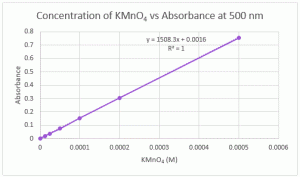Equation and R2 values not containing (0, 0) as a data point: y = 1506.9x + 0.0021 R2 = 1
 Figure 8. Concentration of KMnO4 vs Absorbance of DPH Solutions at λ=530 nmEquation and R2 values not containing (0, 0) as a data point: y = 1506.9x + 0.0021 R2 = 1
 Figure 9. Concentration of KMnO4 vs Absorbance of DPH Solutions at λ=550 nm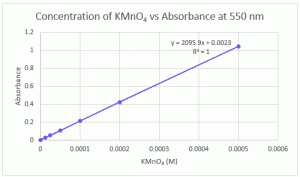Equation and R2 values not containing (0, 0) as a data point: y = 1506.9x + 0.0021 R2 = 1

#### Discussoion

When using spectrophotometry to analyze an API, the UV scan of the API must be taken into account. If the chosen wavelength is at the maximum point of the curve on the compound’s UV scan, this favors linear-type standard curves. If the chosen wavelength is on the sloped shoulders of the UV scan, this favors curved-type standard curves. This is because a slight change in the API’s concentration would result in a greater difference in the absorbance reading.

Figures 10 and 11 show the UV absorbance profile for DPH and KMnO4, respectively.

Figure 10. Absorbance profile of DPH.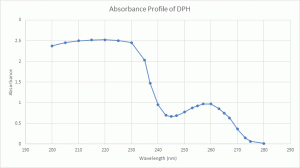Figure 11. Absorbance profile of KMnO4 (Wikipedia).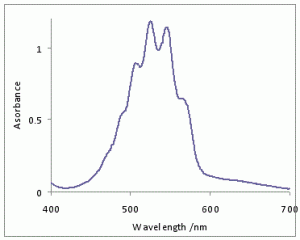The following explanation was taken from “Optical Methods of the Analysis”

#### Limitations to Beer’s Law

According to Beer’s law, a calibration curve of absorbance versus the concentration of analyte in a series of standard solutions should be a straight line with an intercept of 0 and a slope of ab or eb. In many cases, however, calibration curves are found to be nonlinear (Figure 10.22).

Figure 10.22. Calibration curves showing positive and negative deviations from Beer’s law.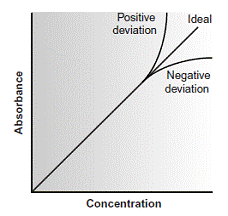#### Fundamental Limitations to Beer’s Law

Beer’s law is a limiting law that is valid only for low concentrations of analyte. There are two contributions to this fundamental limitation to Beer’s law. At higher concentrations the individual particles of analyte no longer behave independently of one another. The resulting interaction between particles of analyte may change the value of e. A second contribution is that the absorptivity, a, and molar absorptivity, e, depend on the sample’s refractive index. Since the refractive index varies with the analyte’s concentration, the values of a and e will change. For sufficiently low concentrations of analyte, the refractive index remains essentially constant, and the calibration curve is linear.

#### Instrumental Limitations to Beer’s Law

There are two principal instrumental limitations to Beer’s law. The first limitation is that Beer’s law is strictly valid for purely monochromatic radiation; that is, for radiation consisting of only one wavelength.

However, even the best wavelength selector passes radiation with a small, but finite effective bandwidth. Using polychromatic radiation always gives a negative deviation from Beer’s law, but is minimized if the value of e is essentially constant over the wavelength range passed by the wavelength selector.

For this reason, as shown in Figure 10.23, it is preferable to make absorbance measurements at a broad absorption peak.

Figure 10.23. Effect of wavelength on the linearity of a Beer’s law calibration curve.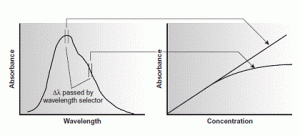In addition, deviations from Beer’s law are less serious if the effective bandwidth from the source is less than one tenth of the natural bandwidth of the absorbing species. When measurements must be made on a slope, linearity is improved by using a narrower effective bandwidth.

Stray radiation is the second contribution to instrumental deviations from Beer’s law. Stray radiation arises from imperfections within the wavelength selector that allows extraneous light to “leak” into the instrument. Stray radiation adds an additional contribution, Pstray, to the radiant power reaching the detector; thus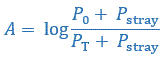For small concentrations of analyte, Pstray is significantly smaller than P0 and PT, and the absorbance is unaffected by the stray radiation. At higher concentrations of analyte, however, Pstray is no longer significantly smaller than PT and the absorbance is smaller than expected. The result is a negative deviation from Beer’s law.

Chemical deviations from Beer’s law can occur when the absorbing species is involved in an equilibrium reaction. Consider, as an example, an analysis for the weak acid, HA. To construct a Beer’s law calibration curve, several standards containing known total concentrations of HA, Ctot, are prepared and the absorbance of each is measured at the same wavelength. Since HA is a weak acid, it exists in equilibrium with its conjugate weak base, A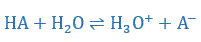If both HA and A absorb at the selected wavelength, then Beer’s law is written as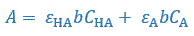where CHA and CA are the equilibrium concentrations of HA and A. Since the weak acid’s total concentration, Ctot, is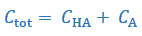the concentrations of HA and A can be written as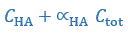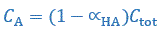where aHA is the fraction of weak acid present as HA. Substituting equations gives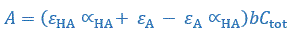Because values of aHA may depend on the concentration of HA, the equation may not be linear. A Beer’s law calibration curve of A versus Ctot will be linear if one of two conditions is met. If the wavelength is chosen such that eHA and eA are equal, then the equation simplifies to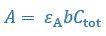and a linear Beer’s law calibration curve is realized. Alternatively, if aHA is held constant for all standards, then equation will be a straight line at all wavelengths.

Because HA is a weak acid, values of aHA change with pH. To maintain a constant value for aHA, therefore, we need to buffer each standard solution to the same pH.

Depending on the relative values of eHA and eA, the calibration curve will show a positive or negative deviation from Beer’s law if the standards are not buffered to the same pH.

#### References

1. Güzelant A, et al. The preventive effect of diphenhydramine on bacterial growth in propofol: a laboratory study. European Journal of Anaesthesiology (2008); 25: 737-740.
2. Optical Methods of the Analysis: Classification of Methods of the Analysis on Chemical, Physical-Chemical, Physical. Available at: http://intranet.tdmu.edu.ua/data/kafedra/internal/pharma_2/classes_stud/en/pharm/prov_pharm/ptn/analytical%20chemistry/2%20course/19%20Optical%20methods%20of%20the%20analysis.htm. Accessed on March 29, 2015.
3. Wikipedia contributors. Vibronic spectroscopy. Wikipedia, The Free Encyclopedia: December 16, 2014, at 15:18 UTC. Available at: http://en.wikipedia.org/wiki/Vibronic_spectroscopy. Accessed March 29, 2015.